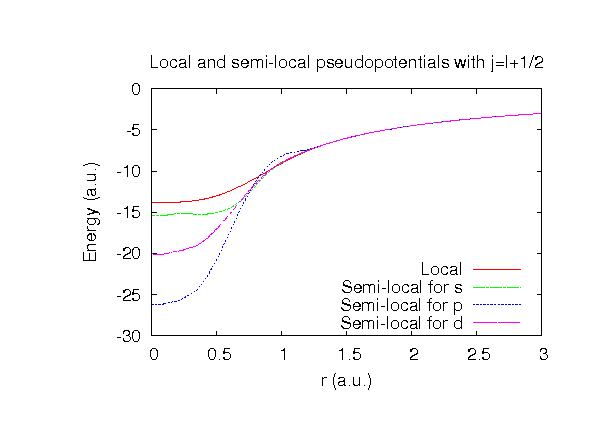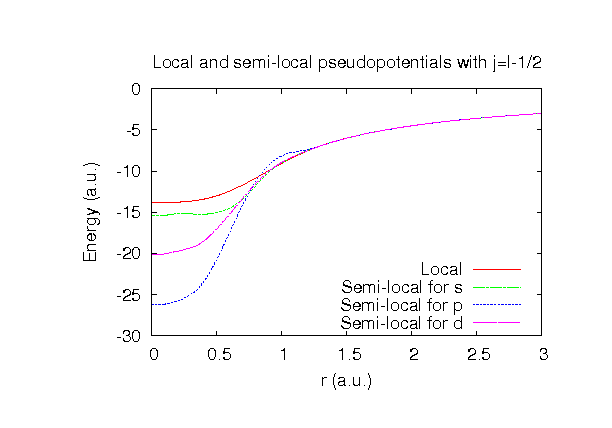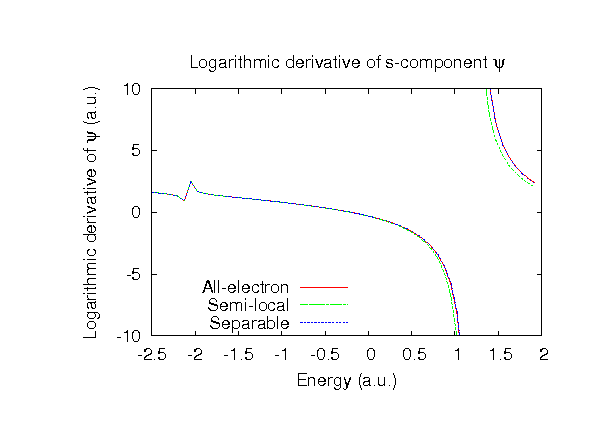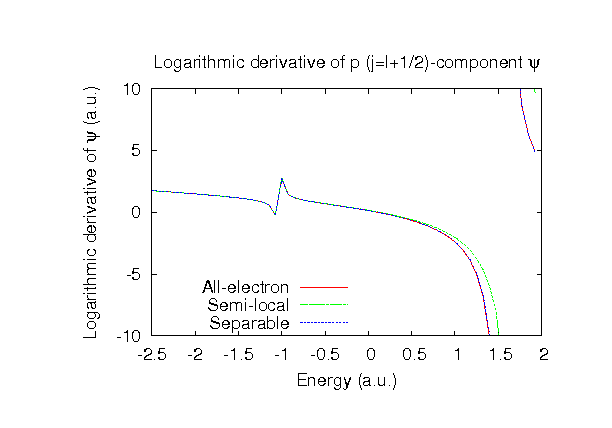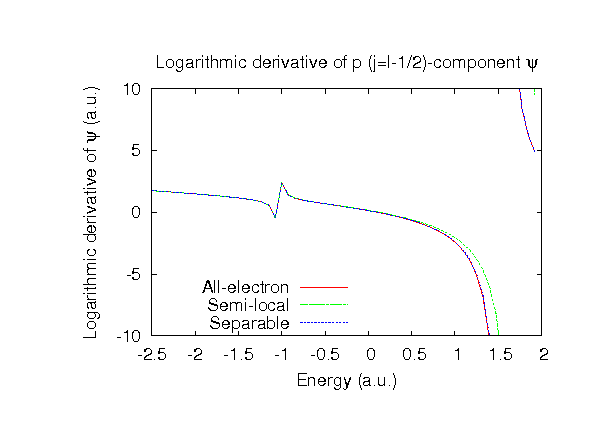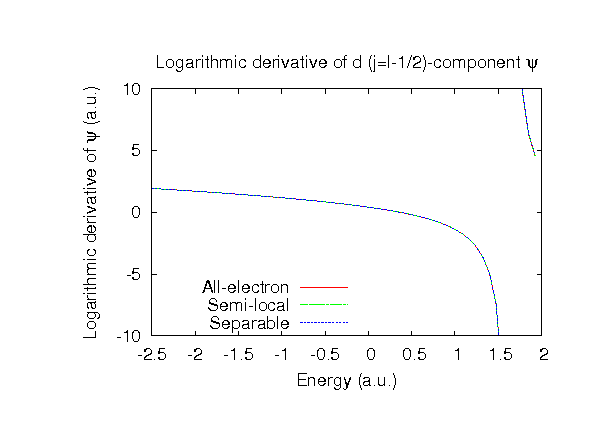### Fully relativistic pseudopotentials

Fully relativistic pseudopotentials generated by the MBK (PRB 47, 6728 (1993)) scheme within LDA (CA13) and GGA (PBE13) which contain a partial core correction and fully relativistic effects including spin-orbit coupling.

### Pseudo-atomic orbitals

The number below the symbol means a cutoff radius (a.u.) of the confinement potential. These file includes fifteen radial parts for each angular momentum quantum number l (=0,1,2,3). The basis functions were generated by variationally optimizing the corresponding primitive basis functions in the single atom and the BCC bulk. The input files used for the orbital optimization can be found at Na_opt.dat and Nabcc_opt.dat . Since Na_CA13.vps and Na_PBE13.vps include the 2s, 2p, and 3s state (9 electrons) as the valence states, the minimal basis set is Na*.*-s2p1. Our recommendation for the choice of cutoff radius of basis functions is that Na9.0.pao is enough for bulks, but Na11.0.pao or Na13.0.pao is preferable for molecular systems.

### Benchmark calculations by the PBE13 pseudopotential with the various basis functions

(1) Calculation of the total energy as a function of lattice constant in the bcc structure, where the total energy is plotted relative to the minimum energy for each case. a0 and B0 are the equilibrium lattice constant and bulk modulus obtained by fitting to the Murnaghan equation of state. The difference between Na9.0-s3p2d1 and Na9.0-s3p3d2 in the total energy at the minimum point is 0.0025 eV/atom. An input file used for the OpenMX calculations can be found at Nabcc-EvsV.dat . For comparison the result by the Wien2k code is also shown, where the calculation was performed by default setting in the Ver. 10.1 of Wien2k except for the use of RMT x KMAX of 12.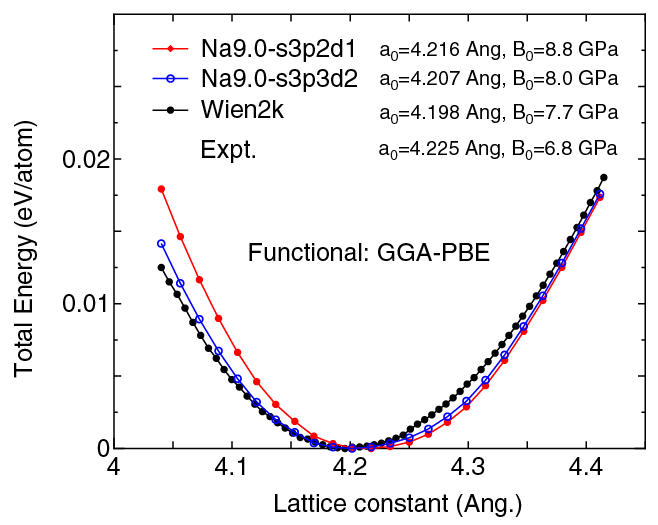(2) Calculations of the band dispersion in the bcc structure, where the non-spin polarized collinear calculation with the lattice constant of 4.225 Ang. was performed using Na_PBE13.vps, Na9.0-s3p2d1, and Na9.0-s3p3d2, and the origin of the energy is taken to be the Fermi level. The input file used for the OpenMX calculations can be found at Nabcc-Band.dat . For comparison the result by the Wien2k code is also shown, where the calculation was performed by default setting in the Ver. 10.1 of Wien2k except for the use of RMT x KMAX of 12.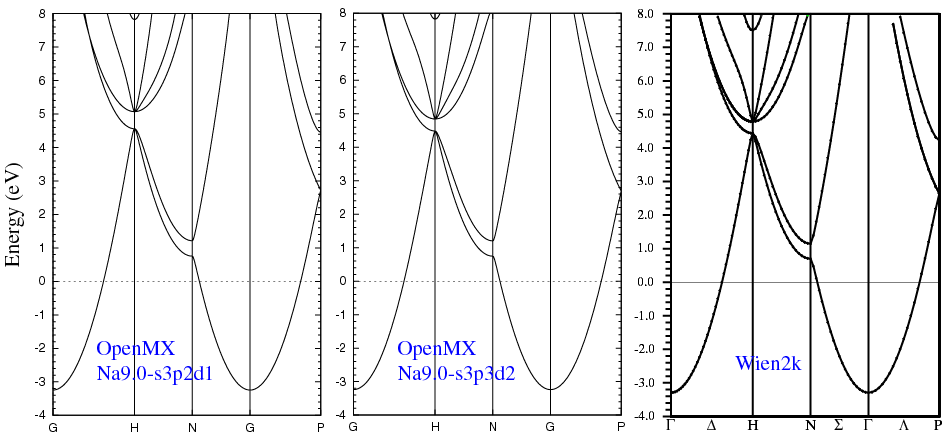(3) Calculations of the total energy as a function of lattice constant of NaF in the rock salt structure, where the total energy is plotted relative to the minimum energy for each case. a0 and B0 are the equilibrium lattice constant and bulk modulus obtained by fitting to the Murnaghan equation of state. The difference between F6.0-s2p2d1 and F6.0-s3p3d2 in the total energy at the minimum point is 0.0044 eV/atom, where Na9.0-s3p2d1 was used as basis functions for Na for both the cases. An input file used for the OpenMX calculations can be found at NaF-EvsV.dat . For comparison the result by the Wien2k code is also shown, where the calculation was performed by default setting in the Ver. 10.1 of Wien2k except for the use of RMT x KMAX of 12.(4) Calculations of the band dispersion of NaF in the rock salt structure, where the non-spin polarized collinear calculation with the lattice constant of 4.62 Ang. was performed using Na_PBE.vps, F_PBE13.vps, Na9.0-s3p2d1, and F6.0-s2p2d1, and the origin of the energy is taken to be the top of the valence band. The input file used for the OpenMX calculations can be found at NaF-Band.dat . For comparison the result by the Wien2k code is also shown, where the calculation was performed by default setting in the Ver. 10.1 of Wien2k except for the use of RMT x KMAX of 12.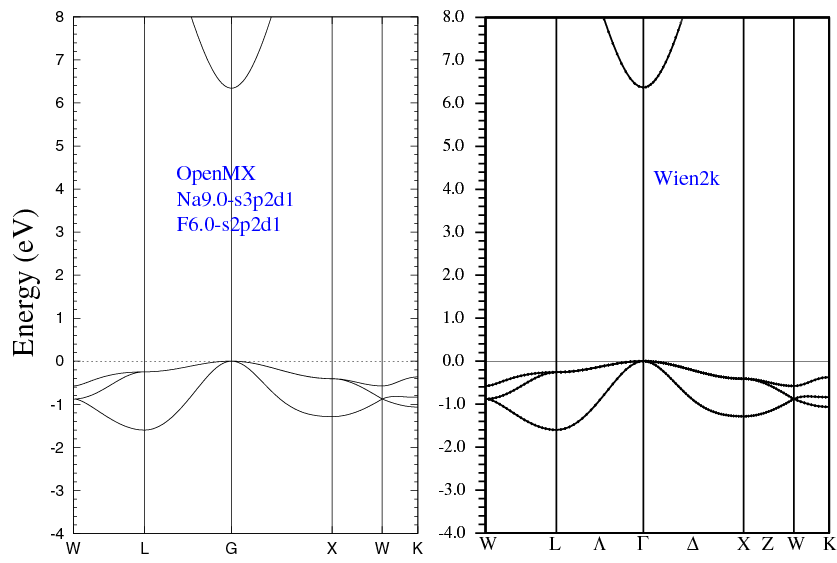(5) Calculations of the total energy as a function of lattice constant of NaCl in the rock salt structure, where the total energy is plotted relative to the minimum energy for each case. a0 and B0 are the equilibrium lattice constant and bulk modulus obtained by fitting to the Murnaghan equation of state. The difference between Cl7.0-s2p2d1 and Cl7.0-s3p3d2 in the total energy at the minimum point is 0.00513 eV/atom, where Na9.0-s3p2d1 was used as basis functions for Na for both the cases. An input file used for the OpenMX calculations can be found at NaCl-EvsV.dat . For comparison the result by the Wien2k code is also shown, where the calculation was performed by default setting in the Ver. 9.1 of Wien2k except for the use of RMT x KMAX of 12. We note that the lattice constant of 5.681 Ang. that we calculated by Wien2k is much smaller than the value (5.714 Ang.) reported in PRB 75, 115131 (2007), although the same functional is used in both the Wien2k calculations.(6) Calculations of the band dispersion of NaCl in the rock salt structure, where the non-spin polarized collinear calculation with the lattice constant of 5.63 Ang. was performed using Na_PBE.vps, Cl_PBE13.vps, Na9.0-s3p2d1, and Cl7.0-s2p2d1, and the origin of the energy is taken to be the top of the valence band. The input file used for the OpenMX calculations can be found at NaCl-Band.dat . For comparison the result by the Wien2k code is also shown, where the calculation was performed by default setting in the Ver. 9.1 of Wien2k except for the use of RMT x KMAX of 12.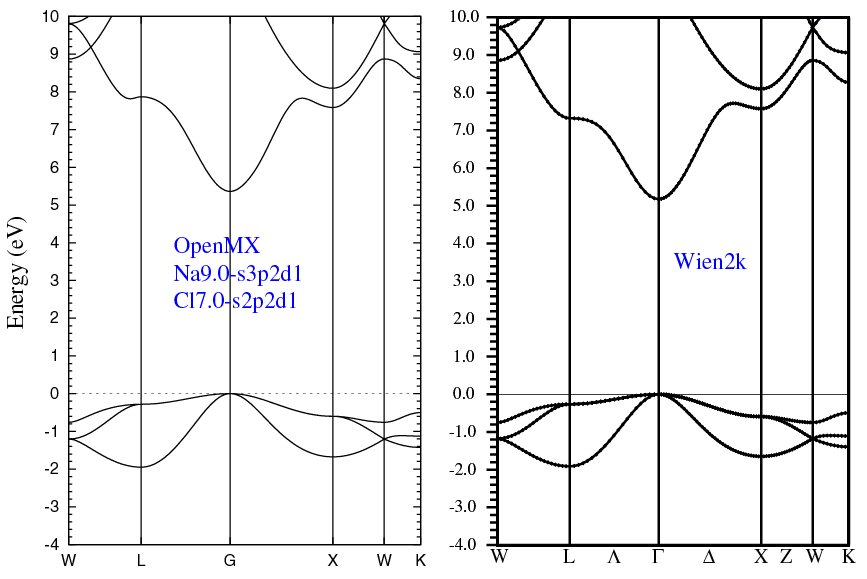(7) Calculations of a sodium molecule with S=1, where Na_PBE13.vps and Na11.0-s3p2d1 or Na11.0-s3p3d2 were used. The input files used for the OpenMX calculations can be found at Na2.dat , Na_cp.dat , and Na.dat .

 Equilibrium bond length (Ang.) Atomization energy (eV) Atomization energy (couterpoise corrected) (eV) Na11.0-s3p2d1 3.081 0.768 0.766 Na11.0-s3p3d2 3.080 0.782 0.776 Other calc. 3.085 a - Expt. 3.079 b 0.747 c

a L. Kronik, I. Vasiliev, and J.R. Chelikowsky, Phys. Rev. B 62, 9992 (2000).
b K.P. Huber and G. Herzberg, Constants of Diatomic Molecules, Van Nostrand Reinhold, New York, 1979.
c K. M. Jones et al., Phys. Rev. A 54, R1006 (1996).

### Supplementary information for the GGA (PBE13) pseudopotential